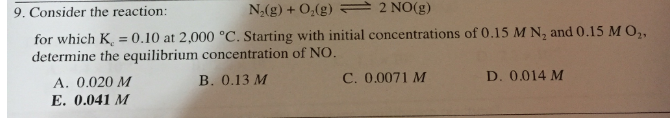# Problem: Consider the reaction: N2(g) + O2(g) ⇌ 2 NO(g) for which Kc = 0.10 at 2,000°C. Starting with initial concentrations of 0.15 M N2 and 0.15 M O2, determine the equilibrium concentration of NO. a. 0.020 M b. 0.13 M c. 0.0071 M d. 0.014 M e. 0.041 M

###### FREE Expert Solution
98% (145 ratings)###### Problem Details

Consider the reaction: N2(g) + O2(g) ⇌ 2 NO(g) for which Kc = 0.10 at 2,000°C. Starting with initial concentrations of 0.15 M N2 and 0.15 M O2, determine the equilibrium concentration of NO.

a. 0.020 M

b. 0.13 M

c. 0.0071 M

d. 0.014 M

e. 0.041 M